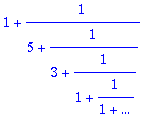> with(numtheory):

```Warning, the protected name order has been redefined and unprotected
```

Pure fifth is 3/2

> w7:=evalf(2^(7/12));> (3/2-w7)/(3/2);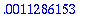> cfrac(w7,4);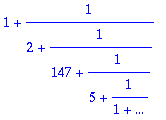Pure fourth is 4/3

> w5:=evalf(2^(5/12));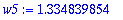> (w5-4/3)/(4/3);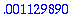> cfrac(w5,4);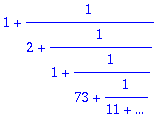Pure major third is 5/4

> w4:=evalf(2^(4/12));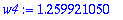> (w4-5/4)/(5/4);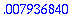> cfrac(w4,4);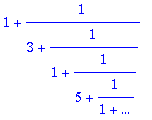Pure minor third is 6/5

> w3:=evalf(2^(3/12));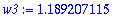> (6/5-w3)/(6/5);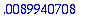> cfrac(w3,4);